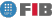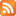# Algebra (AL)

Credits Dept. Type Requirements
9.0 (7.2 ECTS) MAT
• Compulsory for DIE
• Compulsory for DCSFW
• Compulsory for DCSYS

## Instructors

 Person in charge: (-) Others: (-)

## General goals

The overall objective of this subject is to introduce students to basic aspects of whole number arithmetic, combinatorics, linear algebra and geometry. Throughout the subject, emphasis is placed on the techniques of mathematical reasoning.

## Specific goals

### Knowledges

1. Knowledge of set operation. Understand the concept, its applications, and types of set.
2. Understand the concept of Euclidian division, its use in calculating the greatest common divisor of two numbers.

Understanding divisibility properties.
3. Learning the concept of congruence. Learning Fermat"s Theorem and the Chinese Remainder Theorem.
4. Learning the concept and the need for demonstrations. Learning various demonstration techniques, for example, induction.
5. Learn the basic principles of counting. Learn the nature of binomial numbers and some of their basic properties.
6. Learn the basic concepts of linear algebra: dependence, linear independence, bases, orthonormality, base changes and application, orthonormalisation. Linear applications.
7. Learn the geometric transformations relevant to graphic applications and 2D and 3D space.

### Abilities

1. Sets and sub-sets. Operating with sets: union, intersection, Cartesian product. Ability to distinguish between various kinds of application.
2. Ability to calculate the largest common denominator (LCD) of arbitrary numbers. Ability to use the Bezout identity to demonstrate divisibility properties.
3. Ability to work with congruences: resolve systems using the Chinese Remainder Theorem, calculation of powers, among other methods.
4. Ability to make simple demonstrations. Ability to demonstrate that a proposition is false.
5. Ability to select items from a set (whether ordered or not), with and without repetition.

Apply binomial numbers to count selections. Use of the binomial formula and its applications.
6. Ability to use linear algebra to solve geometric and geometric construction problems. Mastery of the vectorial method.
7. Ability to use similar geometric transformations to solve geometric construction problems.

### Competences

1. Ability to apply the techniques for constructing logical/mathematical demonstrations.
2. Ability to transform informal problems into formal ones and vice versa.
3. Learn how to understand problems: given a problem, distinguish between data (or starting elements), unknowns (or what is asked for), hypotheses, and the laws applicable.
4. Ability to think in abstract terms. Ability to tackle new problems by consciously using strategies that have proved useful in solving previous problems.
5. Ability to act independently: Know how to work on one"s own with just the bare minimum of knowledge and guidance.
6. Ability to organise one"s own work: ability to set priorities for various tasks, plan one"s time, and organise one"s notes.
7. Ability to study various sources, recognise that the information obtained in class is insufficient, and to seek the supplementary information required.
8. Ability to learn on one"s own.
9. Ability to present a well-written report setting out one"s results and document submission of practical work).
10. Ability to effectively convey one"s ideas in writing.
11. Knowledge of a foreign language

## Contents

Estimated time (hours):

 T P L Alt Ext. L Stu A. time Theory Problems Laboratory Other activities External Laboratory Study Additional time

1. Preliminaries
T      P      L      Alt    Ext. L Stu    A. time Total
6,0 6,0 0 0 0 10,0 0 22,0
Propositional Logic. Sets. Applications. Successions.

2. Arithmetic
T      P      L      Alt    Ext. L Stu    A. time Total
6,0 6,0 0 0 0 10,0 0 22,0
• Laboratory
Integers and division. Modular arithmetic.

3. Mathematical reasoning
T      P      L      Alt    Ext. L Stu    A. time Total
6,0 6,0 0 0 0 10,0 0 22,0
Demonstration methods. Induction principle.

4. Basic combinational arithmetic
T      P      L      Alt    Ext. L Stu    A. time Total
4,0 4,0 0 0 0 8,0 0 16,0
Basic principles of counting. Permutations and combinations with and without repetition. Binomial numbers.

5. Linear algebra and geometry.
T      P      L      Alt    Ext. L Stu    A. time Total
20,0 20,0 0 0 0 40,0 0 80,0
Vectoral spaces. Linear applications and matrices. Metric problems. Geometric transformations.

 Total per kind T P L Alt Ext. L Stu A. time Total 42,0 42,0 0 0 0 78,0 0 162,0 Avaluation additional hours 6,0 Total work hours for student 168,0

## Docent Methodolgy

Theory classes will take the form of lectures.

Problem classes will be of a participative nature.

## Evaluation Methodgy

Continuous evaluation:

Assessment will be based on:

a part exam (P) (only the first part)
a class exam (E) (only intermediate part)
and a final exam (F) (only on the final part)

The final grade will be calculated in accordance to the following formula:

N=N=0.3P+0.25E+0.45F

Non continuous evaluation:

The final examination consists of the part F (45%) and an extra part EXT (55%), covering the first and intermediate part of the subject.

It is possible to decide if this non continuous evaluation is applied at the moment of the final examination (F). If any exercise of part EXT is solved, non continuous avaluation will be choosen. Resignment of continuous avaluation can not be partial.

Any attempt of fraud during the course will entail the application of the UPC's general academic normative and the beginning of a disciplinary process.

## Basic Bibliography

• Kenneth H. Rosen Discrete mathematics and its applications, McGraw-Hill, 2007.
• Ralph P. Grimaldi Matemáticas discreta y combinatoria : una introducción con aplicaciones, Addison-Wesley Iberoamericana, 1997.
• Howard Anton Introducción al álgebra lineal, Limusa, 2003.

## Complementary Bibliography

• Norman L. Biggs Matemática discreta, Vicens-Vives, 1994.
• Kenneth P. Bogart Matemáticas discretas, Limusa, 1996.
• Eric Lengyel Mathematics for 3D game programming and computer graphics, Charles River Media, 2004.
• Theodore Shifrin, Malcolm R. Adams Linear algebra : a geometric approach, W.H. Freeman, 2002.
• Joan Trias Pairó Geometria per a la informàtica gràfica i CAD, Edicions UPC, 1999.

1. http://www-ma2.upc.es/algebra

## Previous capacities

Basic notions of sets and their application.

Ability to work with inequalities:

Knowledge of integers and the properties of operations.

Operating with matrices: sum, multiply, invert. Ability to calculate the rank of a matrix and its determinants.

Ability to resolve linear systems using the Gauss-Jordan method.

Ability to find equations for R^2 i R^3 straight-lines and planes. Recognise the relative positions between straight lines and R^3 planes. Solving basic 2D and 3D metric problems.

Ordinary scalar product and vectorial product. Module.© Barcelona school of informatics - Contact -RSS
This website uses cookies to offer you the best experience and service. If you continue browsing, it is understood that you accept our cookies policy.
Classic version Mobile version